Journal topic
Proc. IAHS, 381, 95–99, 2019
https://doi.org/10.5194/piahs-381-95-2019Proc. IAHS, 381, 95–99, 2019
https://doi.org/10.5194/piahs-381-95-2019Post-conference publication 01 Aug 2019

Post-conference publication | 01 Aug 2019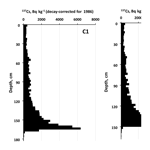# Reconstruction of long-term dynamics of Chernobyl-derived 137Cs in the Upa River using bottom sediments in the Scheckino reservoir and semi-empirical modelling

Reconstruction of long-term dynamics of Chernobyl-derived 137Cs in the Upa River using bottom sediments in the Scheckino reservoir and semi-empirical modelling
Alexei V. Konoplev1, Maxim M. Ivanov2, Valentin N. Golosov2,3,4, and Evgenyi A. Konstantinov4 Alexei V. Konoplev et al.
• 1Institute of Environmental Radioactivity, Fukushima University, Fukushima, 960-1296, Japan
• 2Faculty of Geography, Moscow State University, Moscow, 119991, Russia
• 3Department is Institute of Environmental Sciences, Kazan Federal University, 420008, Kazan, Russia
• 4Institute of Geography, Russian Academy of Science, Moscow, 119017, Russia

Correspondence: Alexei V. Konoplev (alexeikonoplev@gmail.com)

Abstract

Two cores of bottom sediments were collected in 2018 to a depth of ∼200 cm in the deepest part of the Scheckino reservoir on the Upa River (9500 km2), Tula region, Russia. This area was severely contaminated by radiocesium (r-Cs) after the Chernobyl accident in 1986. The fact that 137Cs activity concentrations in a specific horizon of the bottom sediments correspond to 137Cs concentrations associated with suspended matter delivered to the reservoir, provides a basis for constructing the dynamics of particulate 137Cs activity concentrations in the Upa River catchment from 1986 to 2017. Over the time since the Chernobyl accident, the particulate 137Cs concentrations have decreased by more than an order of magnitude, with only minor changes occurring during the last 15 years. Using a typical value for the distribution coefficient Kd for the rivers of the Chernobyl contamination zone, dissolved 137Cs activity concentrations in the Upa River have been estimated and their changes over the past 30 years since the accident have been studied. The resulting estimates of dissolved 137Cs concentrations in the Upa River have been found to be in good agreement with measured data over the period 1987–1991. The proposed and tested method provides a basis for reconstructing the long-term dependence of radionuclide concentrations in rivers and reservoirs based on their vertical distribution in bottom sediments. Reconstructed time dependencies of particulate and dissolved 137Cs activity concentrations in the Upa River were found to be described well by the proposed semi-empirical “diffusion” model based on an assumption that the time dependency of particulate r-Cs in the river corresponds to the time dependency of its concentration in top soil layers across the catchment which can be approximated by a dispersion-convection equation with physically meaningful parameters.

1 Introduction

Large areas of land were contaminated with radionuclides from the Chernobyl fallout (Izrael, 1998). These areas became long-term sources of radionuclides to natural waters and aquatic ecosystems in the Central European Russian landscape (Ivanov et al., 2017). Wash-off by surface runoff is the primary pathway for contamination of water bodies after initial fallout deposition from the atmosphere (Konoplev et al., 1992). Initial radioactive contamination of water bodies after the Chernobyl accident was relatively high as a result of direct fallout onto river and reservoir surfaces.

Over longer time periods after fallout, radionuclides bound in catchment surface soils are slowly transferred to rivers by the erosion of soil particles (particulate phase) and by desorption from soil to solution (dissolved phase). The rates of radionuclide transfer are influenced by the extent of soil erosion, the strength of radionuclide binding to catchment soils and the potential for migration down the soil profile (Golosov et al., 2018). Detailed analysis of Chernobyl data over an extended period provides a basis for long-term prediction of changes in environmental radioactive contamination as a result of the Fukushima accident, or any other emergency involving radioactive emissions to the surrounding environment.

Unfortunately, long-term data are not always available regarding changes in radioactive contamination, and this is the case for 137Cs in the Chernobyl zone rivers. Against this background, this study attempted to reconstruct, from 1986 to the present day, changes in 137Cs concentrations in the Upa River as a result of the Tula-Oryol contamination zone, based on the current vertical distribution of 137Cs (as of 2018) in bottom sediments of the Scheckino reservoir. These changes are described by a semi-empirical diffusion model.

2 Materials and methods

## 2.1 Sampling and processing of bottom sediments

The Upa River basin lies in the northern part of the Central Russian Upland within the Tula region (Fig. 1). Mean annual precipitation in the basin is ∼540 mm yr−1. The southern part of the basin, with a high proportion of arable land, was heavily contaminated by the Chernobyl fallout in April–May 1986. The northern forested part appeared to be less contaminated. Tula city is located in the north-east part of the Upa basin. The upper part of the Upa river (1362 km2) is restricted by the Scheckino reservoir.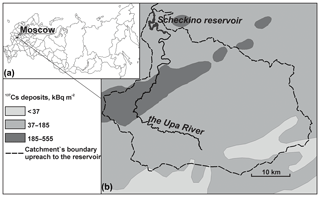Figure 1Location of the Upa river basin (a) and map of Chernobyl-derived 137Cs deposition in the Upa River catchment upstream the Scheckino reservoir (Izrael, 1998) decay corrected for 1 May 1986 (b).

The reservoir has an area of 6 km2 and is elongated upwards of the dam following the drowned valley of the Upa River. For the investigation of radionuclide concentrations in bottom sediments, the upper part of the reservoir was selected since local sources of sediment such as bank erosion and inputs resulting from the transport of sediment by small streams were judged to be minor contributors.

The bottom sediments were collected in February 2018 using a pistol sampler in the deepest parts of the Scheckino reservoir in which higher rates of sedimentation were expected. The cores were wrapped in cellophane film and transported to laboratory. In laboratory, the cores were sliced into 2 cm sections, weighed, dried at 105 C for 8 h and weighed again for determining moisture content and calculating dry sediment mass. The samples were then ground, sieved through 2 mm mesh and placed in a container of defined geometry for measurement of 137Cs activity using a semi-conductor γ-spectrometer with high purity Ge detector.

## 2.2 Semi-empirical “diffusional” model of 137Cs dynamics in river water

### 2.2.1 Particulate 137Cs in surface runoff and rivers

Over the long term, it can be assumed that a major source of suspended matter in surface runoff and rivers is the upper layer of catchment soils. Radionuclide concentrations in top soil decrease over time due to vertical migration to deeper layers. Hence vertical migration in catchment soils is a critical process responsible for the decline in particulate-associated radionuclide concentrations in surface waters. According to the convection-diffusion model (Crank, 1975; Prokhorov, 1981; Bulgakov et al., 2002) a change in radionuclide concentration in the top soil layer Cs with time is given by the following equation:

$\begin{array}{}\text{(1)}& {C}_{\mathrm{s}}\left(t\right)=\frac{\mathit{\sigma }}{\sqrt{\mathit{\pi }{D}_{\mathrm{eff}}t}}{e}^{-\left(\frac{{v}^{\mathrm{2}}}{\mathrm{4}{D}_{\mathrm{eff}}}+\mathit{\lambda }\right)t}\end{array}$

where Deff is the effective dispersion coefficient; v is the effective velocity of convective transport; λ is the radioactive decay constant; σ is the radionuclide deposition density, and t is time.

The time dependence of particulate radionuclide concentrations Cp in surface runoff and river water can be described by this equation using averaged values of Deff and v over the catchment area.

Studies of r-Cs vertical migration in soils of river catchments show that, as a rule, its transport due to dispersion prevails over its convective transport (Konshin, 1992; Ivanov et al., 1997; Konoplev et al., 2016). Therefore,

$\begin{array}{}\text{(2)}& \frac{{v}^{\mathrm{2}}}{\mathrm{4}{D}_{\mathrm{eff}}}\ll \mathit{\lambda }\end{array}$

Eq. (1) can be simplified as follows:

$\begin{array}{}\text{(3)}& {C}_{\mathrm{p}}\left(t\right)=\frac{\mathit{\sigma }}{\sqrt{\mathit{\pi }{D}_{\mathrm{eff}}t}}{e}^{-\mathit{\lambda }t}\sim \frac{{e}^{-\mathit{\lambda }t}}{\sqrt{t}}\end{array}$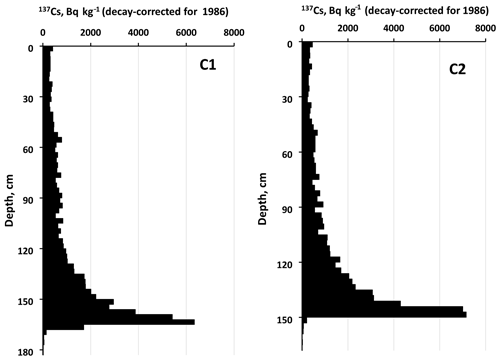Figure 2Vertical distribution of 137Cs, decay-corrected for 1986, in the reservoir cores. The total inventory of 137Cs in core C1 is 1030 kBq m−2 compared with 1160 kBq m−2 in core C2.

### 2.2.2 Dissolved 137Cs in surface runoff and rivers

Dissolved radionuclides in surface runoff and rivers are transferred from soil to water due to cation exchange. As this takes place, only the exchangeable fraction of radionuclides is involved in exchanges with the dissolved phase. Therefore, dissolved radionuclide concentrations in water Cd relate to particulate concentrations as follows (Konoplev and Bulgakov, 2000):

$\begin{array}{}\text{(4)}& {C}_{\mathrm{d}}\left(t\right)=\frac{{C}_{\mathrm{p}}\left(t\right)\cdot {\mathit{\alpha }}_{\mathrm{ex}}}{{K}_{\mathrm{d}}^{\mathrm{ex}}}=\frac{{C}_{\mathrm{p}}\left(t\right)}{{K}_{\mathrm{d}}^{\mathrm{tot}}}\end{array}$

where αex is the exchangeable fraction of the radionuclide, and ${K}_{\mathrm{d}}^{\mathrm{tot}}$ and ${K}_{\mathrm{d}}^{\mathrm{ex}}$ are, respectively, the total and exchangeable distribution coefficients of the radionuclide.

Over longer time periods, when equilibrium between exchangeable and nonexchangeable forms of r-Cs is reached and when condition (2) is valid, the equation for radionuclide dissolved concentrations can be presented as follows:

$\begin{array}{}\text{(5)}& \begin{array}{rl}{C}_{\mathrm{d}}\left(t\right)& =\frac{{\mathit{\alpha }}_{\mathrm{ex}}\left(\mathrm{\infty }\right)\mathit{\sigma }}{{K}_{\mathrm{d}}^{\mathrm{ex}}\sqrt{\mathit{\pi }{D}_{\mathrm{eff}}t}}{e}^{-\mathit{\lambda }t}\\ & =\frac{\mathit{\sigma }}{{K}_{\mathrm{d}}^{\mathrm{tot}}\sqrt{\mathit{\pi }{D}_{\mathrm{eff}}t}}{e}^{-\mathit{\lambda }t}\sim \frac{{e}^{-\mathit{\lambda }t}}{\sqrt{t}}\end{array}\end{array}$

The advantage of this semi-empirical “diffusion-based approach” is that all phases after the accident can be described by the same equation using the same physically-based parameters which can be estimated or determined in the field or using laboratory studies. Generally speaking, the decay-corrected particulate and dissolved 137Cs activity concentrations in both surface runoff and rivers should follow the inverse square root of the time function.

3 Results and discussion

Figure 2 presents the vertical distribution of 137Cs activity concentrations in the two bottom sediment cores collected from the study reservoir (C1 and C2). Accumulation of sediments entering the reservoir is associated with an increase in the total inventory of 137Cs. In both cores, the 137Cs inventory is more than twice the corresponding maximum inventory for the Upa River basin (Fig. 2)

Regretfully, no systematic long-term monitoring of radioactive contamination has been conducted on the Upa River. Data are only available for the first few years after the accident (1987–1991) for total activity concentrations of 137Cs in water (dissolved + particulate) on the Upa River (Vakulovsky et al., 1994). The data obtained by the present study regarding the vertical distribution of 137Cs in bottom sediments in deep-water zones, where sediments tend to accumulate, can provide a basis for reconstructing temporal changes in both particulate and dissolved 137Cs concentrations in the Upa River water following the Chernobyl accident. Here, we make an assumption that annual sediment accumulation on the bottom of the reservoir was not changing over the years since the accident. The shape of the 137Cs vertical distribution in the cored sediment accumulation zones (Fig. 2) indicates that depositing sediments do not mix vertically and that the 137Cs incorporated in them does not migrate, since the 137Cs peak is well defined. Considering the mean rate of sediment accumulation is about 5 cm yr−1, based on the position of the peak radionuclide concentration (Fig. 2), the bottom sediment layers can be attributed to a certain period of sediment accumulation. If 137Cs activity concentration in a respective layer matches its concentration on suspended matter in this time period, changes in 137Cs concentrations on suspended matter in the Upa River from 1986 to 2017 can be easily derived.

Figure 3 shows a reconstructed time dependence of particulate 137Cs concentration in the Upa river, based on the data for the two bottom sediment cores collected in February 2018. As can be seen, over the time since the accident, 137Cs concentrations have decreased by more than an order of magnitude, with only minor changes during the last 15 years.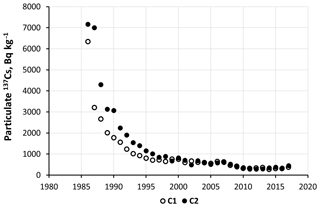Figure 3Reconstructed time dependencies of particulate 137Cs activity concentrations (decay-corrected for 1986) in the Upa River based on 137Cs vertical distributions in cores C1 and C2 collected in February 2018.

Figure 4 shows the results of calculating temporal changes in 137Cs concentrations in suspended material in the Upa River by the described model (line) with concentrations reconstructed based on the sediment core C1. As can be seen, the “diffusion” model provides a good representation of the time dependency of particulate 137Cs concentrations in the Upa River reconstructed using the depth profile in the bottom sediments.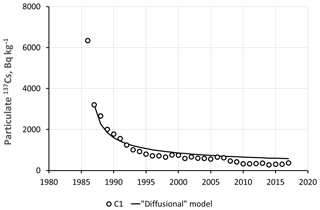Figure 4Diffusion based semi-empirical modelling of reconstructed particulate 137Cs in the Upa River (line) based on the depth profile for the bottom sediments in core C1 (points).

Further, using the typical value of the distribution coefficient Kd for the rivers of the Chernobyl contaminated zone, it is possible to estimate the concentration of dissolved 137Cs in the Upa River and its variation over the 30 years following the accident. A Kd of 20 000 L kg−1 was taken as being a representative value on the basis of analysis of data for a number of rivers in Chernobyl contaminated areas (Konoplev, 2015). Using this value, and reconstructed data for particulate 137Cs activity concentrations, it is possible to estimate the concentrations of dissolved 137Cs in the Upa River for the time window from 1986 to 2017. The results of these estimations are presented in Fig. 5. The published measurement data for 1987–1991 (Vakulovsky et al., 1994) is also given for comparison. It can be seen that the predicted activity concentrations of dissolved 137Cs in the Upa River and their temporal trend are consistent with the measured data.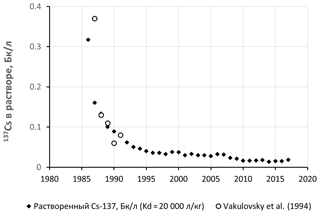Figure 5Comparison of the predicted time dependence of dissolved 137Cs activity concentrations in the Upa River based on reconstructed particulate 137Cs from sediments in core C1 with published measurements for 1987–1991 (Vakulovsky et al., 1994). To calculate dissolved 137Cs activity concentrations, the total distribution coefficient ${K}_{\mathrm{d}}^{\mathrm{tot}}=\mathrm{20}\phantom{\rule{0.125em}{0ex}}\mathrm{000}$ L kg−1 (Konoplev, 2015) was used.

Data availability
Data availability.

The data can be accessed by request to authors.

Author contributions
Author contributions.

AVK suggested the idea and semi-empirical model, MMI, VNG and EAK designed the sampling and provided radiocesium analysis, AVK analyzed the data and prepared the manuscript with contributions from all co-authors.

Competing interests
Competing interests.

The authors declare that they have no conflict of interest.

Special issue statement
Special issue statement.

This article is part of the special issue “Land use and climate change impacts on erosion and sediment transport”. It is a result of the ICCE Symposium 2018 – Climate Change Impacts on Sediment Dynamics: Measurement, Modelling and Management, Moscow, Russia, 27–31 August 2018.

Financial support
Financial support.

This research has been supported by Russian Foundation for Basic Research (RFBR project no. 18-55-50002) and the Japan Society for the Promotion of Science (JSPS) within the framework of the bilateral project “Assessment and prediction of sediment and radionuclide fluxes in a river basin affected by a severe accident”.

References

Bulgakov, A. A., Konoplev A. V., Kanivets, V. V., and Voitsekhovich, O. V.: Modelling the long-term dynamics of radionuclides in rivers, Radioprotection – Colloques, 37, 649–654, 2002.

Crank, J.: The mathematics of diffusion, Oxford University Press, 56–61, London, UK, 1975.

Golosov, V. N., Walling, D. E., Konoplev, A. V., Ivanov, M. M., and Sharifullin, A. G.: Application of bomb- and Chernobyl-derived radiocaesium for reconstructing changes in erosion rates and sediment fluxes from croplands in areas of European Russia with different levels of Chernobyl fallout, J. Environ. Radioactiv., 186, 78–89, https://doi.org/10.1016/j.jenvrad.2017.06.022, 2018.

Ivanov, M. M., Golosov, V. N., and Belyaev, V. R.: Analysis of topography structure for evaluation of sediment delivery ratio within the Plava river basin (Tula oblast), Moscow University Bulletin, ser. 5, Geography, 3, 14–23, 2017.

Ivanov, Y. A., Lewyckyj, N., Levchuk, S. E., Prister, B. S., Firsakova, S. K., Arkhipov, N. P., Kruglov, S. V., Alexakhin, R. M., Sandalls, J., and Askbrant, S.: Migration of 137Cs and 90Sr from Chernobyl fallout in Ukrainian, Belarussian and Russian soils, J. Environ. Radioactiv., 35, 1–21, 1997.

Izrael, Y. A. (Ed.): Atlas of radioactive contamination of European part of Russia, Belarus and Ukraine, Roshydromet, Roskartography, Moscow, Russia, 142 pp., 1998 (in Russian).

Konoplev, A. V.: Distribution of radiocesium of accidental origin between the suspended matter and solution in rivers: Comparison of Fukushima and Chernobyl, Radiochemistry, 27, 552–556, 2015.

Konoplev, A. V. and Bulgakov, A. A.: Exchangeable distribution coefficient of 90Sr and 137Cs in soil-water system, Atom. Energy+, 88, 158–163, 2000.

Konoplev, A. V., Bulgakov, A. A., Popov, V. E., and Bobovnikova, T. I.: Behaviour of long-lived Chernobyl radionuclides in a soil–water system, Analyst, 117, 1041–1047, 1992.

Konoplev, A. V., Golosov, V., Laptev, G., Nanba, K., Onda, Y., Takase, T., Wakiyama, Y., and Yoshimura, K.: Behavior of accidentally released radiocesium in soil-water environment: looking at Fukushima from a Chernobyl perspective, J. Environ. Radioactiv., 151, 568–578, https://doi.org/10.1016/j.jenvrad.2015.06.019, 2016.

Konshin, O. V.: Mathematical model of 137Cs migration in soil: analysis of observations following the Chernobyl accident, Health Phys., 63, 301–306, 1992.

Prokhorov, V. M.: Migration of radioactive contaminants in soils: physico-chemical mechanisms and modelling, Energoatomizdat, Moscow, Russia, 99 pp., 1981 (in Russian).

Vakulovsky, S. M., Nikitin, A. I., Chumichev, V. B., Katrich, I. Y., Voitsekhovich, O. V., Medinets, V. I., Pisarev, V. V., Bovkun, L. A., and Khersonsky, E. S.: Cesium-137 and strontium-90 contamination of water bodies in the areas affected by releases from the Chernobyl nuclear power plant accident: an overview, J. Environ. Radioactiv., 23, 103–122, 1994.